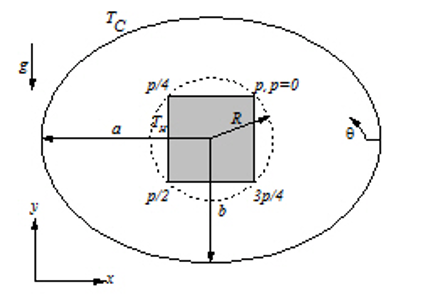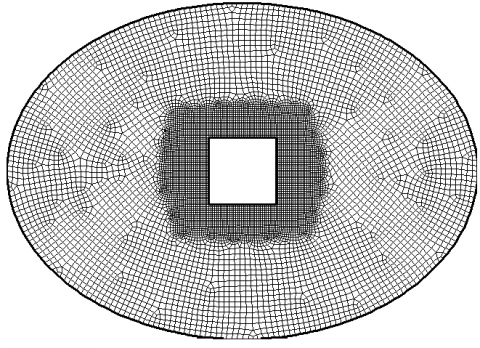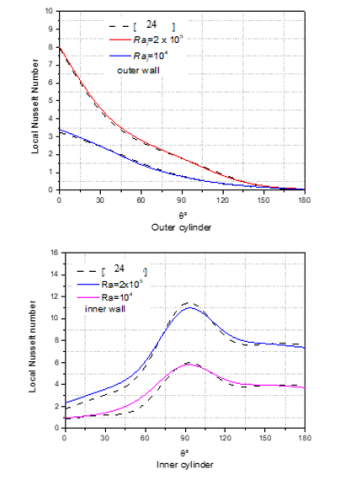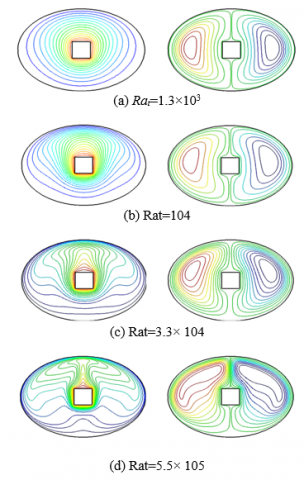# Natural convective heat transfer from a heated horizontal elliptical cylinder to its coaxial square enclosure

Natural convective heat transfer from a heated horizontal elliptical cylinder to its coaxial square enclosure

Abdelkarim BourasDjedid Taloub Mahfoud Djezzar Zied Driss

Department of Physics, Faculty of Sciences, University Mohamed Boudiaf of M’sila, M’sila 28000, Algeria

Laboratory of Energetic Physics, University brothers Mentouri Constantine1, Constantinel, Algeria

Laboratory of Renewable Energy and Sustainable Development (LRESD), University brothers Mentouri Constantinel 25000, Algeria

Laboratory of Electromechanical Systems (LASEM), National School of Engineers of Sfax (ENIS), University of Sfax, Sfax 3038, Tunisia

Corresponding Author Email:
karimbouras200600@gmail.com
Page:
379-385
|
DOI:
https://doi.org/10.18280/mmep.050415
15 October 2018
|
Accepted:
26 November 2018
|
Published:
31 December 2018
| Citation

OPEN ACCESS

Abstract:

A numerical study of the natural convection of laminar heat transfer in the stationary state in a horizontal annular space between a heated square inner cylinder and a cold elliptical outer cylinder was investigated. This annular space is traversed by a Newtonian and incompressible fluid. The Prandtl number is set to 0.71 (air case) for different Rayleigh numbers. The governing equations of the problem were solved numerically by the commercial code Fluent, based on the finite volume method and the Boussinesq approximation. The inner and outer surfaces are isothermal. The study was performed for Rayleigh numbers ranging from 1.3 × 103 to 5.5× 105. Particularly, we have studied the effects of different thermal Rayleigh numbers on natural phenomenon convection. The results were presented in the form of isotherms, streamlines, and local and average Nusselt numbers. The purpose of this study is to observe the influence of the thermal Rayleigh number on the structure of the flow and distribution of the temperature.

Keywords:

natural convection, thermal Rayleigh number, Boussinesq approximation, elliptic-square

1. Introduction

Throughout The study of the natural convection in closed enclosures was the subject of many theoretical and experimental studies. Many published works were elaborated and concern the natural convection in annular spaces such as the square, the rectangle, the triangle, the cylindrical, the elliptic and the spherical geometry.

The considered applications heighted the thermal insulation, the heat exchangers, the cooling of the electronic systems, electrical machines, geophysics, and the nuclear reactors. For example, Calcagni et al.  studied experimentally the free convection in a two-dimensional rectangular cavity with a heating located on the bottom wall through two vertical sides. The upper wall was maintained adiabatic. Lakhal et al.  studied numerically the transient natural convection in a square cavity subjected from below to a sinusoidal variation of the temperature for a Prandtl number equal to 0.71 (air) and for Rayleigh numbers varying from 105 to 106. Hossain and Wilson  studied unsteady laminar natural convection in a rectangular enclosure formed by non-isothermal walls. The walls horizontal top and vertical rights of the enclosure are cooled. The bottom wall was heated to a constant temperature, whereas the left vertical wall was heated but it was considered non-isothermal for Rayleigh number equal to 105. Kazmierczak et al.  studied the transient natural convection in a square cavity due to a sinusoidal variation of the temperature in a hot vertical wall. The opposite wall (cold) was maintained at a constant temperature constantly lower than that of the vertical wall. They analyzed the effects of the period and the amplitude of the oscillating temperature through the cold wall of the cavity. All transient solutions were obtained in a periodic time. Ridouane et al.  studied numerically the laminar natural convection in a triangular cavity filled with air. The vertical walls were heated and the inclined walls were cooled. The variations of the Nusselt number were correlated according to the Rayleigh number and the angle of inclination (α compressed in the range of 5° to 63°). Also, the authors compared this rate of transfer with that of the differentially heated cavity. They show that this rate is very important for low Rayleigh and falls for the strong values of Rayleigh. Chamkha and Ismael  studied numerically the heat transfer convection-conduction in a square cavity heated by triangular solid wall in steady-state. The governing equations of the heat transfer were based on the Darcy model. They analyzed the effect of the nanofluid type, the voluminal fraction in nanoparticles, the Rayleigh number, the dimension of the obstacle and the thermal conductivity compared to the base fluid, on the characteristics of flow and heat transfer. Kholal et al.  studied numerically the mixed convection in a circular tube inclined to the horizontal and heated using a heat flow constant and uniform on its entire circumferential surface. The flow inside the tube was laminar. The governing elliptic differential equations were solved using the finite volume method and for a Reynolds number equal to 500 and different combinations of the Grashof number (104, 105, 106) and angle of inclination (0°, 30°, 60°, 90°). Francis et al.  made a study on natural convection in a space between two concentric cylinders. They used a CFD calculation code to reproduce the experimental results. In this comparative study, the interest was mainly concentrated on large widths (of the order of half a meter or more) and large radius ratios. The steady-state CFD analysis consisting of concentric cylindrical models for larger geometries was compared favorably with the results of correlations of Kuehn and Goldstein [9-10] in the Rayleigh number varying between 105 and 108. Khaleel and Dawood  examined numerically the influence of the installation of two adiabatic obstacles on the natural convection in a porous layer of constant thickness with different lengths and angular positions. This porous layer surrounded by two elliptic cylinders, isothermal hot surface for the inner wall and isothermal cold surface for the outer wall. Ghernoug et al. [12-13] studied numerically the phenomenon of the natural convection, in laminar and permanent in an annular space, situated between two horizontal eccentric cylinders, oriented according to an angle α. They considered in a first case, both cylindrical isothermal walls of the enclosure. In a second case, the inner cylindrical wall was submitted to a density flux constant. The effect of inclination, relative eccentricity values and Grashof number on the results obtained was examined. Bouras et al. [14-15] investigated double diffusive natural convection in an annular space between two elliptical cylinders of confocal horizontal axes. They used thermal Rayleigh numbers up to 5x105. Also, they examined the influence of Rayleigh number and Prandtl number on isotherms, is concentrations and streamlines. Mehrizi et al. [16-17] studied numerically the laminar natural convection by the method of Lattice Boltzmann, in two-dimensional annulus between a heated triangular inner cylinder and an elliptical outer cylinder. A constant temperature limit condition was imposed on both the inner and outer surfaces. The study was performed for different angles of inclination of the inner triangular and outer elliptical cylinders. The space is filled with air as a working fluid. Mehrizi et al.  studied the effect of nanoparticles on the heat transfer by natural convection. Xu et al.  studied numerically the heat transfer by transitory natural convection of gallium between two coaxial cylinders in a circular cylinder on the outside and a triangular cylinder inside, for a Prandtl number equal to 0.023.

According to these anterior results, it is clear that the study of the natural convection has been studied by different authors. However, the square ellipse enclosure has evoked considerable interest; it arises from available works very little information is currently available.

In the present study, we are interested in the natural convection in an annular space delimited by two cylinders of horizontal axes, heated by a square inner cylinder and a cold elliptical outer cylinder. This annular space is traversed by a Newtonian and incompressible fluid, in steady-state and laminar. Particularly, we studied the effect of the Rayleigh number on the structure of the flow, the distributions of the temperature and the current function. Thus the rates of heat transfer are presented by the local and average Nusselt numbers.

2. Physical Model Mathematical Formulation

2.1 Description of the problem

Figure 1 shows a cross section of the system. In this system, we consider the elliptical annular space characterized by the eccentricity of the outer elliptical tube (e = 0.7). The inner cylinder presents a heated square characterized by the dimension h, outer cylinder presents a cold elliptic. The annular space is closed and contains an incompressible fluid with a kinematic viscosity ν=1,50.10-5 and thermal diffusivity α= 2,08.10-5. The outer wall and the inner wall generate a vertical gradient in temperature (active walls).

## 1.pngFigure 1a. Schematic presentation of the physical model

## 2.pngFigure 1b. A typical grid distribution (230×130) with uniform and orthogonal distributions

2.2 Simplifying hypotheses

The flow of natural convection is caused by the thermal forces of pushes and remains laminar. It is supposed that the physical properties are constant except for the density of the mixture which depends on its temperature according to the relation of the approximation of Boussinesq:

$\rho(T)=\rho_{0}\left[1-\beta_{t}\left(T-T_{0}\right)\right]$ (1)

2.3 Mathematical formulation

The characteristic quantities used to adimension the problem are: the temperature differences ΔT = Tc-Tf  between the walls of the system and the thermal diffusivity α of the fluid.

The mathematical model includes the continuity equation, the momentum equations and energy equation.

The equation of continuity com is written as follows:

$\frac{\partial U}{\partial X}+\frac{\partial V}{\partial y}=0$ (2)

The momentum equations are written as follows:

$U \frac{\partial U}{\partial X}+V \frac{\partial U}{\partial Y}=-\frac{\partial P}{\partial X}+\frac{\partial^{2} U}{\partial X^{2}}+\frac{\partial^{2} U}{\partial Y^{2}}$ (3)

$U \frac{\partial V}{\partial X}+V \frac{\partial V}{\partial Y}=-\frac{\partial P}{\partial Y}+\frac{\partial^{2} V}{\partial X^{2}}+\frac{\partial^{2} V}{\partial Y^{2}}+\frac{R a}{\operatorname{Pr}} \theta$ (4)

The equation of energy is written as follows:

$U \frac{\partial \theta}{\partial X}+V \frac{\partial \theta}{\partial Y}=\frac{1}{\operatorname{Pr}}\left(\frac{\partial^{2} \theta}{\partial X^{2}}+\frac{\partial^{2} \theta}{\partial Y^{2}}\right)$  (5)

The initial condition is:

$U=V=0$  (6)

$T=T_{0}=T_{F}$  (7)

In addition, the boundary conditions on the system are defined in the internal cylinder (trapeze) as follows:

$\mathrm{u}=\mathrm{v}=0$  (8)

$\mathrm{T}=\mathrm{T}_{\mathrm{C}}$ (9)

However, in the external cylinder (ellipse), we can write:

$\mathrm{u}=\mathrm{v}=0$  (10)

$T=T_{F}$   (11)

The dimensionless parameters that govern the problem are the thermal Rayleigh (Rat), Prandtl number (Pr) and Nusselt numbers (Nu).

The thermal Rayleigh number for this problem is defined by:

$R a_{T}=\frac{g \beta\left(T_{C}-T_{F}\right) H^{3}}{\alpha v}$  (12)

where H is the characteristic length, which is chosen as the space between the two cylinders. The number of Prandtl is defined as follows:

$\operatorname{Pr}=\frac{v}{\alpha}$ (13)

The local Nusselt number is defined on the characteristic length L by:

$N u_{l}=L\left.\frac{\partial \Phi}{\partial \vec{n}}\right|_{W a l l}$  (14)

where $\vec{n}$ is a normal vector on the wall, and ф is the dimensionless temperature. The perimeter of the ellipse Pe and the perimeter of square P were chosen as the characteristic length L.

The average Nusselt number for the ellipse and the square can be evaluated by:

$\overline{N}_{u_{o}}=\frac{1}{P_{e}} \int_{0}^{P} N u_{L} d P e$  (15)

$\overline{\mathrm{Nu}}_{\mathrm{s}}=\frac{1}{\mathrm{P}} \int_{0}^{\mathrm{P}} \mathrm{Nu}_{\mathrm{L}} \mathrm{d} \mathrm{P}$  (16)

The average Nusselt number for both surfaces is:

$N u_{a g}=\frac{\overline{N} u_{e}+\overline{N} u_{s}}{2}$  (17)

2.4 Meshing choice

In this study, several grids were used arbitrarily for the considered configuration (Figure 1b) for Rat=105 to observe their effect on the results. Table 1 shows, therefore, the variation of the average Nusselt number, according to the considering nodes. According to these simulations the grid 230 X 130 appears more adequate to choose.

Table 1. Variation of the average Nusselt number according to the number of nodes for Rat=105

 MMesh size 200x100 210x110 220x120 230x130 240x140 NNu (avg) 5.6496 5.5818 5.4955 5.4319 5.4250 RRelative EError (%) 1.2147       1.5704       1.1709         0.1272

2.5 Numerical method

The governing equations are solved iteratively by employing the control volume method. The commercial CFD code Fluent 6.3 was used as a solver to study the natural convection heat transfer, with the imposed boundary conditions. This method has the advantage of satisfying the mass, the momentum and energy equations in all the volumes. The computational domain was generated and meshed in the environment of the preprocessing code Gambit 2.3. Fine structured quadrilateral cells are generated in the thin boundary layers near the walls and grid structured not uniform tight are generated near to the borders of the walls. The spatial term in the governing equations are discretized using the Body Force Weighted implicit scheme. This type of scheme was recommended for the flows implying of important voluminal forces. The second-order scheme was used since it allows some stability and minimizes the numerical diffusion. The SIMPLE algorithm of Patankar and Spalding  was used to implement the pressure-velocity coupling. In addition, the residuals of calculation were controlled to ensure the convergence criterion and the stability of the solution.

3. Results and Discussion

The effect of different Rayleigh numbers on the natural convection of heat transfers in an enclosure delimited by two horizontal confocal cylinders elliptic and square, filled of air (Pr = 0.71) was studied for four different Rayleigh numbers. Results are presented in the form streamlines, isotherms, local and average Nusselt numbers.

## 3.pngFigure 2. Streamline (left half) and isotherms (right half) to Rat = 104

## 4.pngFigure 3. Local Nusselt number along inner and outer ellipses

The natural convection between the horizontal elliptic cylinders confocal by ElShamy et al.  was chosen for the validation of this study. These results were presented in the form of isotherms and of streamlines for two different Rayleigh numbers, as shown in figure 2. Moreover, the local Nusselt number was compared with the results of ElShamy  for different Rayleigh numbers extending from 104 to 105 (figure 3). In the case of two elliptic horizontal confocal, the eccentricity of the inner and outer wall was taken 0.9 and 0.4, respectively, and the Rayleigh number equal to 104 (figure 2). The local Nusselt numbers of the inner and outer cylindrical ellipse for two Rayleigh numbers based on original paper description were plotted in figure 3. The result indicates an acceptable agreement with the results which presented by ElShamy et al. . In every case, the results show that two symmetrical cells of recirculation are formed in right and left of the vertical symmetry of the cavity. It due to the strength of buoyancy produces by gradient of temperature. In these conditions, the fluid is raised on the side of inner cylinder, and the flow becomes fresh and denser by the outer cylinder.

3.1 Effect of the thermal Rayleigh number

Figure 4 shows the isotherms and streamlines for different values of the thermal Rayleigh number equal to $R a_{t}=1.310^{3}, \mathrm{Ra}_{\mathrm{t}}=10^{4}, R a_{t}=3.310^{4}$ and $R a_{t}=5.510^{5} .$.

For the thermal Rayleigh number equal to 1.3 103 (figure 4(a)), the isotherms are concentric around the square. As for streamlines, it has been observed that the flow is organized in two cells which turn very slowly in opposite directions, one to the right and the other to the left of the inner wall. The two cells are similar in shape and symmetrical to a vertical line passing through the center of the square. The values of the streamlines are very small. The process of heat transfer takes place mainly by conduction because the convection is weak. The buoyancy force is proportional to the difference in temperature being very low.

## 5.pngFigure 4. Isotherms and streamlines

In the case of the thermal Rayleigh number equal to 104 (figure 4(b)), the isotherms change substantially to follow the direction of flow. Also, the values of the streamlines on the same figure increase significantly, reflecting a transformation from conductive to convective transfer. The hot fluid in the vicinity of the square tube rises under the effect of buoyancy and the cold fluid near the elliptical cylinder falls under the effect of gravity.

For Rat = 3.3×104 (figure 4(c)), the isotherms with the top of the square tube change the hemispherical form to the shape of thermal plume. The ascending flow appears monocellulaire on the side of the cylinder interior and going down on the side of the cylinder external. In this case, the natural convection predominates and the values of the streamlines increase. At higher Rayleigh.

Rat = 5.5×105 (figure 4(d)), the boundary layers become thinner and longer. The centers of the recirculation cells move upwards because the convection effects become greater. The heat transfer rate and the value of the streamlines are higher. So, we find that the intensity of the flow increases with the growth of the Rayleigh number

3.2 Local Nusselt number along the outer

Figure 5 illustrates the evolution of the local Nusselt number on the wall of the elliptical cylinder. According to these results, it is clear that the increase of the Rayleigh number increases the value of the local Nusselt number. For a small Rayleigh number Rat = 1.3×103, this increase is small since most heat transfer is conductive. For the largest values of the Rayleigh number (Rat = 3.3×104 and 5.5×105), the maximum of the local Nusselt number is reached at the angular position θ = 90°. However, the minimum value is located in the lower part of the elliptical cylinder located at θ=270 °. In this range of Rayleigh numbers, most of the heat transfer is by convection, as we have deduced from figure 4.

## 5a.pngFigure 5. Variation of the local Nusselt number along the outer wall

3.3 Local Nusselt number along the inner wall

Figure 6 presents the variation of the local Nusselt numbers along the inner walls. According to these results, it has been noted that the increase in the thermal Rayleigh number, the value of the local Nusselt number increases. The Nusselt number is important in the corners of the square tube and is low on the rest of the square.Indeed, it has been observed that the local Nusselt number is larger on the two upper corners, where the heat transfer rate is important compared to two bottom corners.

## 6.pngFigure 6. Variation of the local Nusselt number along the inner wall

3.4 Average Nusselt number

## 7.pngFigure 7. Variation of the average Nusselt number according to the thermal Rayleigh number

Figure 7 illustrates the variation of the average Nusselt number as a function of the thermal Rayleigh number. According to these results, it is clear that at low values of the thermal Rayleigh number (Rat≤104), the Nusselt number values are constant and almost close to the conductive value. So, the heat transfer is essentially conductive when the Rayleigh number is small as we have deduced from figure 4. For the greatest values of the thermal Rayleigh number, the rate of the thermal transfer increases with the increase of the thermal Rayleigh number. Then, the transfer of the heat becomes convective.

4. Conclusions

In this paper, we have numerically studied the laminar and permanent two-dimensional natural convection in an annular space between two cylinders. The inner cylinder is square in shape and the outer cylinder is elliptical, filled with a Newtonian and incompressible fluid.

The mathematical model describing the present problem was developed based on the continuity equation, the Navier-stokes equations and the energy equation. We have presented the effect of the thermal Rayleigh number on the flow structure, the temperature distributions and the streamline as well as the heat transfer rates represented respectively by the local and average Nusselt numbers.

The obtained results confirm that for a small thermal Rayleigh number, the heat transfer within the annulus is essentially controlled by the conduction process. As the thermal Rayleigh number increases (Rat ≥104), the role of convection becomes predominant. The local and average Nusselt numbers increase with the increase of the thermal Rayleigh number. The local Nusselt number is important in the corners of the square wall (bigger on the two upper corners) and small on the rest of the latter. On the hot wall, the maximum of the local Nusselt number is reached at the angular position (θ = 90 °), and the minimum is reached in the lower elliptical cylinder (θ = 270 °).

These results will be used for the design of engineering and the process improvement of the heat exchangers, the drying processes, the cooling of electronic circuits and the cooling of nuclear reactors.

Nomenclature

a       rand axe de cylindre elliptique

b       Petit axe de cylindre elliptique

Nuavg        Nombre de Nusselt moyen

h       Longueur de la carré, m

$\overline{Nu}_{ellipse}$  Nombre de Nusselt locol externe

$\overline{Nu}_{carré}$   Nombre de Nusselt locol externe

Pr     Nombre de Prandtl

e       Excentricité de l'ellipse

Rat   Nomber de Rayleigh thermique

P      Pression au sein du fluide

∆T    Ecart de température, K

Tf     Température de la paroi froide externe, K

Tc     Température de la paroi chaude externe, K

Lettres grecques

α    Diffusivité thermique,m2/ s

β    Coefficient d’expansion

ν    Viscosité cinématique, m2/S

θ       Angle

ρ       Masse volumique du fluide, kg/m3

References

 Calcagni B, Marsili F, Paroncini M. (2005). Natural convective heat transfer in square enclosures heated from below. Applied Thermal Engineering 25: 2522–2531.

 Lakhal EK, Hasnaoui M. (1995). Convection naturelle dans une cavité carrée chauffée  périodiquement par le bas. Revue générale de thermique 27: 480-485.

 Hossain MA, Wilson M. (2002). Natural convection flow in a fluid-saturated porous medium enclosed by non-isothermal walls with heat generation. International Journal of Thermal Sciences 41: 447-454.

 Kazmierczak M, Chinoda Z. (1992). Buyabcy-driven flow in an enclosure with time periodic boundary conditions. International Journal of Heat and Mass Transfer 32(6): 1507-1519.

 Ridouane EH, Campo A, Chang JY. (2005). Natural convection patterns in right-angled triangular cavities with heated vertical sides and cooled hypotenuses. Journal of Heat Transfer 127: 1181-1186.

 Chamkha AJ, Ismael MA. (2013). Conjugate heat transfer in a porous cavity filled with nanofluid and heated by a triangular thick wall. Int. J. Therm. Sci 67: 135-151.

 Kholal O, Bellaouar A, kadja M. (2007). Etude numérique de la convection mixte dans un tube incliné. 13èmesJournées Internationales de Thermique.

 Francis DN. (2002). CFD calculation of internal natural convection in the annulus between horizontal concentric cylinders. Reported by United States Department of Energy by Sandia Corporation, SAND 2002-3132.

 Kuehn TH, Goldstein RJ. (1976). An experimental and theoretical study of natural convection in the annulus between horizontal concentric cylinders. J. Fluid Mech 74: 695-719.

 Kuehn TH, Goldstein RJ. (1976). Correlating equations for natural convection heat transfer between horizontal circular cylinders. Int. J. Heat Mass Transfer 19: 1127-1134.

 Khaleel FA, Dawood AS. (2014). The effect of adiabatic obstacles on natural convection inside a horizontal elliptical porous annulus. Mechanical Engineering Research 4(1).

 Ghernoug C, Djezzar M, Bouras A. (2013). The natural convection in annular space located between two horizontal eccentric cylinders: The Grashof number effect. Energy Procedia 36: 293-302.

 Gernoug C, Djezzar M, Naji H, Bouras A. (2016). Towards numerical computation of double-diffusive natural convection within an eccentric horizontal cylindrical annulus. Int. J. of Numerical Methods for Heat & Fluid Flow (Ed. Emerald).

 Bouras A, Djezzar M, Naji H, Ghernoug C. (2014). Numerical computation of double-diffusive natural convective flow within an elliptic-shape enclosure. International Communications in Heat and Mass Transfer 57: 183-192.

 Bouras A, Djezzar M, Ghernoug C. (2013). Numerical simulation of natural convection between two elliptical cylinders: Influence of Rayleigh number and Prandtl number. Energy Procedia 36: 788-797.

 Mehrizi A, Mohamad A. (2015). Effect of the inclination angle and eccentricity on free convection heat transfer in elliptical–triangular annuli: A lattice boltzmann approach, numerical heat transfer, part A: Applications. An International Journal of Computation and Methodology 68.

 Mehrizi AA, Sedighi K, Farhadi M, Sheikholeslami M. (2013). Lattice Boltzmann simulation of natural convection heat transfer in an elliptical-triangular annulus. International Communications in Heat and Mass Transfer 48: 164-177.

 Mehrizi AA, Farhadi M, Shayamehr S. (2013). Natural convection flow of Cu–Water nanofluid in horizontal cylindrical annuli with inner triangular cylinder using lattice Boltzmann method. Int. Commun. Heat Mass Transf 44: 147-156.

 Xu X, Yu Z, Hub Y, Fan L, Cen K. (2012). Transient natural convective heat transfer of a low-Prandtl-number fluid from a heated horizontal circular cylinder to its coaxial triangular enclosure. International Journal of Heat and Mass Transfer 55: 995-1003.

 Patankar S, Spalding D. (1972). A calculation procedure for heat, mass and momentum transfer in three-dimensional parabolic flows. Int. J. heat and Mass transfer 15: 1787-1806.

 El Shamy MM, Ozisik MN, Coulter JP. (1990). Correlation for laminar natural convection between confocal horizontal elliptical cylinders, Numer. Heat Transfer, Part A 18: 95-112.